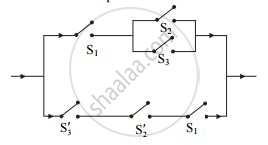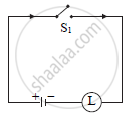# Construct the Simplified Circuit for the Following Circuit - Mathematics and Statistics

Construct the simplified circuit for the following circuit:#### Solution

Let p : switch S1 is closed
q : switch S2 is closed
r : switch S3 is closed
~ p : switch S1 is open
~ q : switch S2 is open
~ r : switch S3 is open
The symbolic form of the given circuit
[p ∧ (q ∨ r)] ∨ [~ r ∧ ~ q ∧ p]
= p ∧ [(q ∨ r) ∨ (~ q ∧ ~ r) ….(Distributive law)
= p ∧ [(q ∨ r) ∨ ~ (q  r)] ….(De Morgan’s law)
= p ∧ T ….(Compliment law)
= p ….(Identity law)
The new simplified circuit isConcept: Application of Logic to Switching Circuits
Is there an error in this question or solution?
2014-2015 (October)

Share